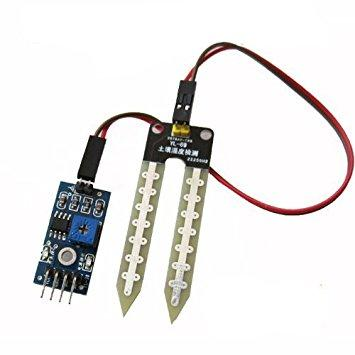# ESP32 and Soil Moisture Sensor example

In this article, we are going to interface a Soil moisture sensor with an ESP8266. This sensor measures the volumetric content of water inside the soil and gives us the moisture level as output. The sensor is equipped with both analog and digital output.

The soil moisture sensor consists of two probes which are used to measure the volumetric content of water. The two probes allow the current to pass through the soil and then it gets the resistance value to measure the moisture value.When there is more water, the soil will conduct more electricity which means that there will be less resistance. Therefore, the moisture level will be higher. Dry soil conducts electricity poorly, so when there will be less water, then the soil will conduct less electricity which means that there will be more resistance. Therefore, the moisture level will be lower.

Input Voltage: 3.3 – 5V
Output Voltage: 0 – 4.2V
Input Current: 35mA
Output Signal: Both Analog and Digital
Pin Out
The  soil moisture sensor  has four pins:

VCC: Power
A0: Analog Output
D0: Digital Output
GND: Ground
The Module also contains a potentiometer which will set the threshold value. This threshold value will be compared by the LM393 comparator. The output LED will light up and down according to this threshold value.

To connect the sensor in the analog mode, we will need to use the analog output of the sensor. When taking the analog output from the soil moisture sensor, the sensor gives us a value from 0 to 1023. The moisture is measured in percentage, so we will map these values from 0 to 100 and then we will show these values on the serial monitor.

Connections

The connections for the soil moisture sensor to the ESP32 LOLIN32 are as follows:

VCC of the sensor to 3v3 of the Wemos
GND of the sensor to GND of the Wemos
A0 of the sensor to 36 of the LOLIN32

Analog Example

```<pre>int sensor_pin = 36;
int value ;

void setup()
{
Serial.begin(9600);
delay(2000);
}

void loop()
{

value = map(value,550,0,0,100);
Serial.print("Moisture : ");
Serial.print(value);
Serial.println("%");
delay(1000);
}</pre>
```

Another example

```<pre>/*
# the approximate moisture levels for the sensor reading
# 0 to 300 dry soil
# 300 to 700 humid soil
# 700 to 950 in water
*/

# define ledPin 15
# define sensorPin 36

int trigger = 300; // set the level

void setup()
{
Serial.begin(9600);
pinMode(ledPin, OUTPUT);
digitalWrite(ledPin, LOW); // turn off LED
}

void loop()
{
Serial.print("Moisture Sensor Value:");
{
digitalWrite(ledPin, HIGH); // turn on the LED
}
else
{
digitalWrite(ledPin, LOW); // turn off LED
}
delay(500);
}</pre>
```

Digital example

To connect the soil moisture sensor in the digital mode, we will connect the digital output of the sensor to the digital pin of the LOLIN32. The Sensor module contains a potentiometer, which is used to set the threshold value. The threshold value is then compared with the sensor output value using the LM393 comparator which is placed on the sensor module.

The LM393 comparator compares the sensor output value and the threshold value and then gives us the output through the digital pin. When the sensor value is greater than the threshold value, the digital pin will give us 5V and the LED on the sensor will light up. When the sensor value will be less than this threshold value, the digital pin will give us 0V and the light will go down.

Connections
The connections for the soil moisture sensor and the ESP32 in digital mode are as follows.

VCC of sensor to 3v3 of ESP32
GND of sensor to GND of ESP32
D0 of sensor to pin 36 of ESP32
LED positive to pin 15 of ESP32
LED negative to GND of ESP32

Code

```<pre>int led_pin =15;
int sensor_pin =36;

void setup()
{
pinMode(led_pin, OUTPUT);
pinMode(sensor_pin, INPUT);
}

void loop()
{
{
digitalWrite(led_pin, HIGH);
}
else
{
digitalWrite(led_pin, LOW);
delay(1000);
}
}</pre>
```